# Undetermined coefficients, method of

(diff) ← Older revision | Latest revision (diff) | Newer revision → (diff)

The determination of an unknown function in the form of an exact or approximate linear combination (finite or infinite) of known functions. This linear combination is taken with unknown coefficients, which are determined in one way or another from the conditions of the problem in question. As a rule, one obtains a system of algebraic equations for them.

A classic example of the method of undetermined coefficients is its use in the expansion of a regular rational function in a complex or real domain in elementary fractions. Letandbe algebraic polynomials with complex coefficients, where the degreeof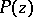is less than the degreeofand the coefficient of the highest term of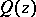is 1, let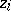be a root ofof multiplicity,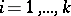,, so that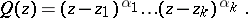The regular rational function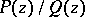can be represented uniquely in the form(1)

where theare yet unknown complex numbers (altogether). To find them, both parts of the equality are brought to a common denominator. After this, by disregarding inessential terms and reduction of similar terms on the right-hand side one obtains an equality on each side of which there stand polynomials of degree at most; on the left-hand side with known coefficients, on the right-hand side in the form of linear combinations of the unknown numbers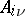. By equating coefficients at equal powers of, one obtains a system oflinear equations in thewhich, owing to the existence and uniqueness of the expansion (1), has a unique solution. Occasionally it is convenient to use a somewhat different device for finding the coefficients. For example, suppose that all roots ofare simple, so that (1) takes the form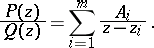After bringing the two sides to a common denominator and reduction of similar terms, one obtains the equality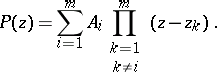When one sets in it in succession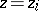,, one readily obtainsIn the general case it is useful to combine these two devices for finding the coefficients.

Let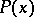andbe polynomials with real coefficients,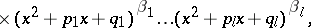where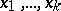are the real roots of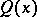of multiplicities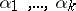, and the quadratic trinomialwith real coefficientsand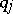is the product, where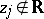is a complex root of multiplicity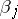,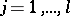, ofand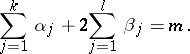Then for the regular rational functionthere is one and only one expansion of the form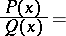(2)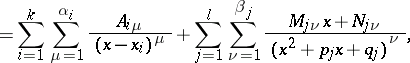where the coefficients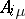,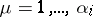,,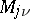and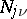,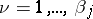,, are real numbers. The method for finding them is the same as in the complex case described above: the equality (2) is brought to a common denominator, inessential terms are disregarded, and after collecting similar terms the coefficients at equal powers ofon both sides are equated. As a result one obtains a system ofequations in theunknowns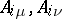, which has a unique solution.

The expansion of regular rational functions into elementary ones is applied, for example, to find their Laurent series (in particular, their Taylor series), and to integrate them. The method of undetermined coefficients is also used to integrate rational functions by means of the Ostrogradski method, and to integrate functions of the form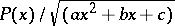. In this case the integral becomes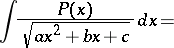(3)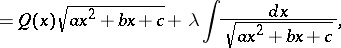where the degree of the polynomialis one less than that of. To find the coefficients ofand the numberone differentiates (3). Then one brings both sides to a common denominator, disregards inessential terms, collects similar terms, and equates coefficients at equal powers of. As a result one obtains again a system of linear equations with a unique solution. Similar methods of integration can be applied in certain other cases.

The method of undetermined coefficients is applied in finding solutions of (ordinary and partial) differential equations in the form of power series. For this purpose, in a neighbourhood of the point in question a power series with undetermined coefficients is substituted in the given equation. Sometimes one obtains as a result relations between the coefficients of the series from which by means of given initial or boundary conditions one succeeds in finding these coefficients and, consequently, a solution of the equation in the form of a series. For example, when one solves the hypergeometric equation in this manner, one can obtain an expansion in a series of hypergeometric functions (cf. Hypergeometric function).

The method of undetermined coefficients is also applied in other ways when solving differential equations, for example, the Galerkin method, the Ritz method and the Trefftz method; it is also used in numerical methods: in Krylov's method for obtaining the coefficients of the secular equation, and in the approximate solution of integral equations.